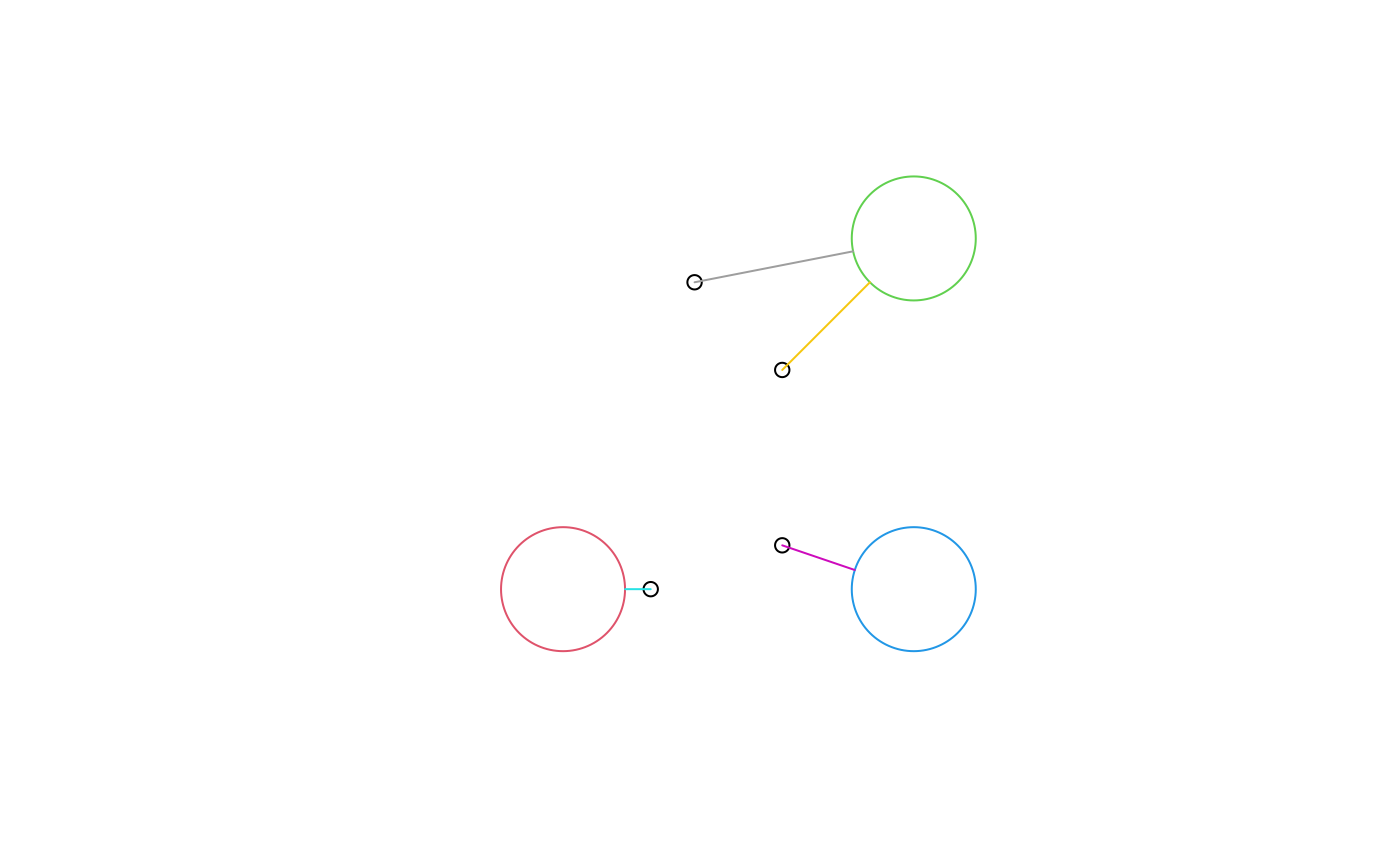get index of nearest feature

## Usage

st_nearest_feature(
x,
y,
...,
check_crs = TRUE,
longlat = isTRUE(st_is_longlat(x))
)

## Arguments

x

object of class sfg, sfc or sf

y

object of class sfg, sfc or sf; if missing, features in x will be compared to all remaining features in x.

...

ignored

check_crs

logical; should x and y be checked for CRS equality?

longlat

logical; does x have ellipsoidal coordinates?

## Value

for each feature (geometry) in x the index of the nearest feature (geometry) in set y, or in the remaining set of x if y is missing; empty geometries result in NA indexes

st_nearest_points for finding the nearest points for pairs of feature geometries

## Examples

ls1 = st_linestring(rbind(c(0,0), c(1,0)))
ls2 = st_linestring(rbind(c(0,0.1), c(1,0.1)))
ls3 = st_linestring(rbind(c(0,1), c(1,1)))
(l = st_sfc(ls1, ls2, ls3))
#> Geometry set for 3 features
#> Geometry type: LINESTRING
#> Dimension:     XY
#> Bounding box:  xmin: 0 ymin: 0 xmax: 1 ymax: 1
#> CRS:           NA
#> LINESTRING (0 0, 1 0)
#> LINESTRING (0 0.1, 1 0.1)
#> LINESTRING (0 1, 1 1)

p1 = st_point(c(0.1, -0.1))
p2 = st_point(c(0.1, 0.11))
p3 = st_point(c(0.1, 0.09))
p4 = st_point(c(0.1, 0.9))

(p = st_sfc(p1, p2, p3, p4))
#> Geometry set for 4 features
#> Geometry type: POINT
#> Dimension:     XY
#> Bounding box:  xmin: 0.1 ymin: -0.1 xmax: 0.1 ymax: 0.9
#> CRS:           NA
#> POINT (0.1 -0.1)
#> POINT (0.1 0.11)
#> POINT (0.1 0.09)
#> POINT (0.1 0.9)
try(st_nearest_feature(p, l))
#>  1 2 2 3
try(st_nearest_points(p, l[st_nearest_feature(p,l)], pairwise = TRUE))
#> Geometry set for 4 features
#> Geometry type: LINESTRING
#> Dimension:     XY
#> Bounding box:  xmin: 0.1 ymin: -0.1 xmax: 0.1 ymax: 1
#> CRS:           NA
#> LINESTRING (0.1 -0.1, 0.1 0)
#> LINESTRING (0.1 0.11, 0.1 0.1)
#> LINESTRING (0.1 0.09, 0.1 0.1)
#> LINESTRING (0.1 0.9, 0.1 1)

r = sqrt(2)/10
b1 = st_buffer(st_point(c(.1,.1)), r)
b2 = st_buffer(st_point(c(.9,.9)), r)
b3 = st_buffer(st_point(c(.9,.1)), r)
circles = st_sfc(b1, b2, b3)
plot(circles, col = NA, border = 2:4)
pts = st_sfc(st_point(c(.3,.1)), st_point(c(.6,.2)), st_point(c(.6,.6)), st_point(c(.4,.8)))
plot(pts, add = TRUE, col = 1)
# draw points to nearest circle:
nearest = try(st_nearest_feature(pts, circles))
if (inherits(nearest, "try-error")) # GEOS 3.6.1 not available
nearest = c(1, 3, 2, 2)
ls = st_nearest_points(pts, circles[nearest], pairwise = TRUE)
plot(ls, col = 5:8, add = TRUE)# compute distance between pairs of nearest features:
st_distance(pts, circles[nearest], by_element = TRUE)
#>  0.05857864 0.17481378 0.28284271 0.36849479